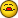# Array to Multiple Arrays

H

#### Howard

Earlier today Claus offered me a simpler version of copy array to an array code.

Here I have embellished it to copy from one array to four arrays.
As below, is this in line with best practices? It works fine as is, however on the fourth array I thought I could use... myArr5 = Array("L5:L12") but it errors out. Seems to want each cell listed in the array. I have seen an example in a google search where...

myArr5 = Array("L5:L12").Select
Selection = myArray

Or something similar.
The code is in a standard Module 1 of the workbook.

Thanks.
Howard

Option Explicit

Dim myarray(8) As Variant
Dim myArr2 As Variant
Dim myArr3 As Variant
Dim myArr4 As Variant
Dim myArr5 As Variant

Dim i As Integer

Sheets("Sheet1").Activate

myarray(0) = Range("F13")
myarray(1) = Range("F16")
myarray(2) = Range("F19")
myarray(3) = Range("I13")
myarray(4) = Range("I16")
myarray(5) = Range("I19")
myarray(6) = Range("L13")
myarray(7) = Range("K17")

Sheets("Sheet2").Activate

myArr2 = Array("F3", "C3", "D3", "I3", "J3", "M3", "O3", "P3")
myArr3 = Array("A3", "B5", "C7", "D9", "E11", "F13", "G15", "H17")
myArr4 = Array("A20", "B20", "C20", "D20", "E20", "F20", "G20", "H20")
myArr5 = Array("L5", "L6", "L7", "L8", "L9", "L10", "L11", "L12")

For i = LBound(myArr2) To UBound(myArr2)

Range(myArr2(i)) = myarray(i)
Range(myArr3(i)) = myarray(i)
Range(myArr4(i)) = myarray(i)
Range(myArr5(i)) = myarray(i)
Next
End Sub

C

#### Claus Busch

Hi Howard,

Am Fri, 14 Jun 2013 11:24:50 -0700 (PDT) schrieb Howard:
myArr5 = Array("L5:L12").Select
Selection = myArray
if you have adjacent cells you don't need an array.
Try:

Dim myarray(7) As Variant
Dim myArr2 As Variant
Dim myArr3 As Variant
Dim i As Integer

With Sheets("Sheet1")
myarray(0) = .Range("F13")
myarray(1) = .Range("F16")
myarray(2) = .Range("F19")
myarray(3) = .Range("I13")
myarray(4) = .Range("I16")
myarray(5) = .Range("I19")
myarray(6) = .Range("L13")
myarray(7) = .Range("K17")
End With

myArr2 = Array("F3", "C3", "D3", "I3", "J3", "M3", "O3", "P3")
myArr3 = Array("A3", "B5", "C7", "D9", "E11", "F13", "G15", "H17")

With Sheets("Sheet2")
For i = LBound(myArr2) To UBound(myArr2)
.Range(myArr2(i)) = myarray(i)
.Range(myArr3(i)) = myarray(i)
Next
.Range("A20").Resize(, 8) = myarray
.Range("L5").Resize(8, 1) = WorksheetFunction.Transpose(myarray)
End With
End Sub

Regards
Claus Busch

H

#### Howard

Hi Howard,

Am Fri, 14 Jun 2013 11:24:50 -0700 (PDT) schrieb Howard:

if you have adjacent cells you don't need an array.

Try:

Dim myarray(7) As Variant

Dim myArr2 As Variant

Dim myArr3 As Variant

Dim i As Integer

With Sheets("Sheet1")

myarray(0) = .Range("F13")

myarray(1) = .Range("F16")

myarray(2) = .Range("F19")

myarray(3) = .Range("I13")

myarray(4) = .Range("I16")

myarray(5) = .Range("I19")

myarray(6) = .Range("L13")

myarray(7) = .Range("K17")

End With

myArr2 = Array("F3", "C3", "D3", "I3", "J3", "M3", "O3", "P3")

myArr3 = Array("A3", "B5", "C7", "D9", "E11", "F13", "G15", "H17")

With Sheets("Sheet2")

For i = LBound(myArr2) To UBound(myArr2)

.Range(myArr2(i)) = myarray(i)

.Range(myArr3(i)) = myarray(i)

Next

.Range("A20").Resize(, 8) = myarray

.Range("L5").Resize(8, 1) = WorksheetFunction.Transpose(myarray)

End With

End Sub

Regards

Claus Busch

Ah Ha! I get it, A20 resize 8 columns for the horizontal and L5 resize 8 rows for the vertical along with the transpose.

Thanks again Claus.

Regards,
Howard

G

#### GS

I'd say what you have here is easy to understand/maintain for the few
target ranges you have in your sample code. Claus' approach is more
efficient.

I'm inclined to populate all cells receiving the source values in one
shot since the sync is the same for all target cells...

Const sRng1\$ = "F13,F16,F19,I13,I16,I19,L13,K17"

Const sRng2\$ = "F3,A3,A20,L5:C3,B5,B20,L63,C7,C20,L7" _
& ":I3,D9,D20,L8:J3,E11,E20,L9:M3,F13,F20,L10" _
& ":O3,G15,G20,L113,H17,H20,L12"

...where sRng2 is a delimited string of delimited strings, the latter
being the target cells to be passed as the range addresses for the
target array as follows...

Sub XferVals2(Optional sSrc\$, Optional sTgt\$)
Dim va1, va2, i%

If sSrc = "" Then sSrc = sRng1: If sTgt = "" Then sTgt = sRng2
va1 = Split(sSrc, ",")
If InStr(1, sTgt, ":") > 0 _
Then va2 = Split(sTgt, ":") _
Else va2 = Split(sTgt, ",")

For i = LBound(va1) To UBound(va1)
Range(va2(i)).Value = Range(va1(i)).Value
Next 'i
End Sub

...so you can now have it both ways<g>!

--
Garry

Free usenet access at http://www.eternal-september.org
Classic VB Users Regroup!
comp.lang.basic.visual.misc
microsoft.public.vb.general.discussion